## An 18.5-cm-diameter loop of wire is initially oriented perpendicular to a 1.5-T magnetic field. The loop is rotated so that its plane is par

Question

An 18.5-cm-diameter loop of wire is initially oriented perpendicular to a 1.5-T magnetic field. The loop is rotated so that its plane is parallel to the field direction in 0.20 s. What is the average induced emf in the loop?

in progress 0
6 months 2021-07-29T02:52:25+00:00 1 Answers 22 views 0

The average induced emf in the loop is 0.20 V

Explanation:

Given:

Radius of loop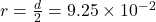m

Magnetic field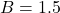T

Change in time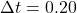sec

Induced emf is given by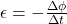Where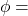magnetic flux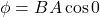( here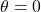)

Where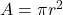We neglect minus sign because it’s shows lenz law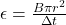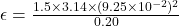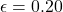V

Therefore, the average induced emf in the loop is 0.20 V